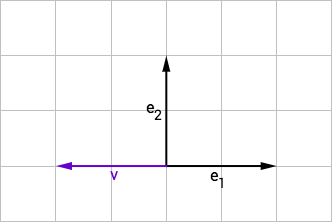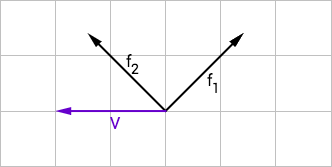# The change of basis matrix

## Change of basis

You can represent a vector $\color{blue}{\vec{v}}$ by its coordinates in a basis. For instance, here are the coordinates for the vector $\vec{v}$ in two different basis $(e) = (e_1, e_2)$ and $(f) = (f_1, f_2)$:

#### Base 1 ($e_1$, $e_2$)$\color{blue}{\vec{v}} = \begin{pmatrix}-1\\0\end{pmatrix}^e = -1 e_1 + 0 e_2$

#### Base 2 ($f_1$, $f_2$)$\color{blue}{\vec{v}} = \begin{pmatrix}-0.5\\+0.5\end{pmatrix}^f = -0.5 f_1 + 0.5 f_2$

### The change of basis matrix

We can use a matrix to transform the coordinates of $\vec{v}$ in the basis $(e)$ into the coordinates in the basis $(f)$. This matrix is called the change of basis matrix.

How do we find this matrix? Since it takes the coordinates of a vector in basis $(e)$ and gives the coordinates in the basis $(f)$, we can start by expressing the vectors $e_1$ and $e_2$ in basis $(f)$.

• In basis $(e)$: $e_1 = \begin{pmatrix}1\\0\end{pmatrix}^e$ and $e_2 = \begin{pmatrix}0\\1\end{pmatrix}^e$

…and…

• In basis $(f)$: $\color{red}{e_1} = \begin{pmatrix}\color{red}{0.5}\\\color{red}{-0.5}\end{pmatrix}^f$ and $\color{green}{e_2} = \begin{pmatrix}\color{green}{0.5}\\\color{green}{0.5}\end{pmatrix}^f$.

So we are looking for the matrix $M_{f\leftarrow e}$ such that:

…and…

Since $M_{f\leftarrow e} \cdot (1, 0)^\top$ is the first column of $M_{f\leftarrow e}$ and $M_{f\leftarrow e} \cdot (0, 1)^\top$ is the second column of $M_{f\leftarrow e}$, we find that the change of basis matrix is the matrix made of the vector column of the old basis $(e)$ expressed in the new basis $(f)$. We note $\vec{v}^f$ the coordinates of vector $\vec{v}$ in base $(f)$.

Using this matrix, we can translate the coordinates for $\color{blue}{\vec{v}}$ from basis $(e)$ to basis $(f)$:

### From vector notation to sum notation

Sometimes, it’s useful to remember that matrix multiplication can be expressed as a sum of vectors using linearity.

The change of basis matrix allows us to write equations where some vectors are expressed in base $(f)$ (left hand side) while others are in base $(e)$ (right hand side).

Using the notation $\vec{v}^f$ for the coordinates of vector $\vec{v}$ in base $(f)$:

Where every vector in the last equation is expressed in base $(f)$.

Other articles you might like: